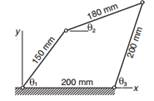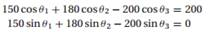Create an Account

Home / Questions / The three angles shown in the figure of the four bar linkage are related by Determine ...

The three angles shown in the figure of the four bar linkage are related by Determine θ 1 and θ 2 when θ 3 75◦ Note that there are two solutionsThe three angles shown in the figure of the four-bar linkage are related byDetermine θ 1 and θ when θ 3 = 75◦. Note that there are two solutions.

Jul 27 2020 View more View LessSubscribe To Get Solution